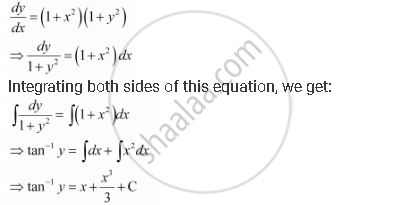Share

# For the Differential Equations Find the General Solution: Dy/Dx = (1+X^2)(1+Y^2) - CBSE (Commerce) Class 12 - Mathematics

ConceptMethods of Solving First Order, First Degree Differential Equations Differential Equations with Variables Separable

#### Question

For the differential equations find the general solution:

dy/dx = (1+x^2)(1+y^2)

#### Solution

The given differential equation is:This is the required general solution of the given differential equation.

Is there an error in this question or solution?

#### APPEARS IN

NCERT Solution for Mathematics Textbook for Class 12 (2018 to Current)
Chapter 9: Differential Equations
Q: 6 | Page no. 396
Solution For the Differential Equations Find the General Solution: Dy/Dx = (1+X^2)(1+Y^2) Concept: Methods of Solving First Order, First Degree Differential Equations - Differential Equations with Variables Separable.
S